Calculating Camera Sensor Resolution and Lens Focal Length

Introduction

First, define these basic terms for your situation:

• Field of view (FOV): Area under inspection that the camera needs to acquire
• Smallest feature: The size of the smallest feature that you want to detect in the image
• Working distance (WD): Distance from the front of the lens to the object under inspection

See the following images for reference: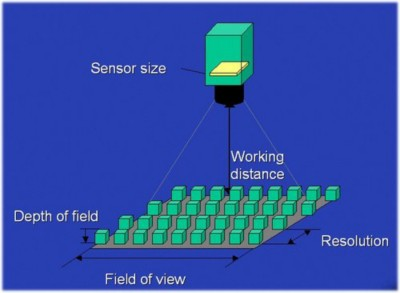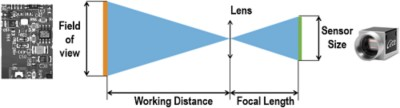Follow two main steps to pick the minimum required camera resolution and to determine the correct focal length for your application.

1. Calculate minimum sensor resolution: Knowing the field of view and smallest feature you want to detect.
2. Select a camera: Once the camera is selected you can use the sensor size to calculate the focal length.

Sensor Resolution

The resolution of an image is the number of pixels in the image. This is in two dimensions; for example 640X480. The calculations can be done for each dimension separately; but, for simplicity, this is often reduced to one dimension.

To make an accurate measurement on the image, you need to use a minimum of two pixels per smallest feature that you want to detect. To do the calculation for the minimum sensor resolution, multiply two (pixels/smallest feature) times the size (in real-world units) of the field of view divided by the size of the smallest feature as shown in the following equation: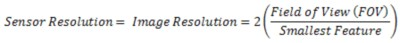Calculate sensor resolution:

• Example 1: My FOV is 100mm and my smallest feature is 1mm. Following the equation, the needed minimum sensor resolution is 200 pixel. A camera with a resolution of 640x480 will work because 200 is less than the smallest dimension which is 480.
• Example 2: My FOV is 500mmX600mm and my smallest feature is 2mm. It is generally assumed that the smallest feature is round with this 2mm diameter. Following the equation, the minimum sensor resolution is 500X600. The sensor of 640X480 will be not sufficient. A sensor resolution of 1024X768 or 1280X1024 would be more appropriate in this case.

The equation can be modified to solve for any other variable so long as three variables are known.

Sensor Size

Sensor size refers to the physical size of the sensor, and is typically not noted on specification sheets. The best way to determine sensor size is to look at the pixel size on the sensor and multiply by the resolution.

Calculate sensor size:

• Example 3: According to the camera user manual the Basler acA1300-30um has a pixel size of 3.75 x 3.75um and a resolution of 1296 x 966 pixels. The sensor size is then 3.75um x 1296 by 3.75um x 966 = 4.86 x 3.62mm.

Sensor Format

Sensor format refers to the physical size of the sensor, but is not dependent on the pixel size. This specification is used to determine what lens the camera is compatible with. In order for a lens to be compatible with a camera, the format of the lens needs to be greater than or equal to the sensor format. If a lens with a smaller format is use, the image experiences vignetting; this causes regions of the sensor outside of the lens format area to be dark.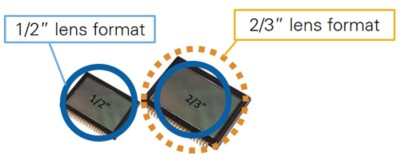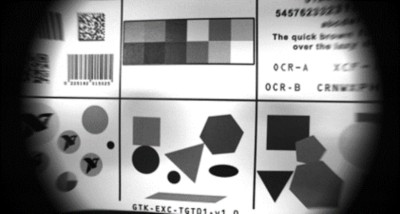Focal Length

Generally, lenses have fixed focal lengths. Also, it is common that the working distance is flexible, so for simple calculations start out with a ratio of working distance to focal length. This will allow you to use specific lens focal lengths to determine the working distance needed. If the working distance is limited, then, by inverting this ratio, we get the ratio of focal length to working distance. This will allow you to use a range of working distance options to get a focal length range. Then once a lens is selected you can recalculate the exact working distance needed.

These calculations are based on the following equation: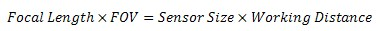Calculate Focal Length:

• Example 4 (Using Flexible working distance): My FOV is 508mm  x 381mm, my sensor size is 8.47mm (diagonal). The ratio of working distance to focal length is 381mm/8.47mm = 45:1. So, if I choose a focal length of 25mm (which is about 1") then a working distance of about 1140mm (45") is required. If the maximum working distance available is 889mm (35"), then inverting the ratio (1:45) gives the maximum focal length of 35/45 = 19.76mm (7/9") so a focal length of 16mm will do. Reversing the calculation gives a required working distance of about 711.2mm (28").
• Example 5 (Using Fixed working distance): My FOV is 609.6mm x 609.6mm, my sensor format is 12.7mm (diagonal) and my working distance is 1016mm. In order to be exact in solving for focal length, you would need to know the aspect ratio of the sensor. If exact is not necessary the diagonal will do. Solving the equation above for focal length will be (12.7X1016)/609.6 = 21.2mm. This is not a common lens focal length so either the working distance would need to be adjusted or a non-standard lens that allows the user to vary the focal length is required.

Lenses are manufactured with a limited number of standard focal lengths. Common lens focal lengths include 6 mm, 8 mm, 12.5 mm, 25 mm, and 50 mm. Once you choose a lens whose focal length is closest to the focal length required by your imaging system, you need to adjust the working distance to get the object under inspection in focus.

Note: Lenses with short focal lengths (less than 12 mm) produce images with a significant amount of distortion. If your application is sensitive to image distortion, try to increase the working distance and use a lens with a higher focal length. If you cannot change the working distance, you are somewhat limited in choosing a lens.

For your Basler camera feel free to use the Basler Lens Selector tool.